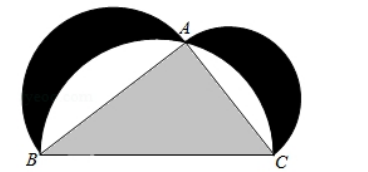$\text{A.}$ $\mathrm{p}_{1}=\mathrm{p}_{2}$ $\text{B.}$ $\mathrm{p}_{1}=\mathrm{p}_{3}$ $\text{C.}$ $\mathrm{p}_{2}=\mathrm{p}_{3}$ $\text{D.}$ $\mathrm{p}_{1}=\mathrm{p}_{2}+\mathrm{p}_{3}$